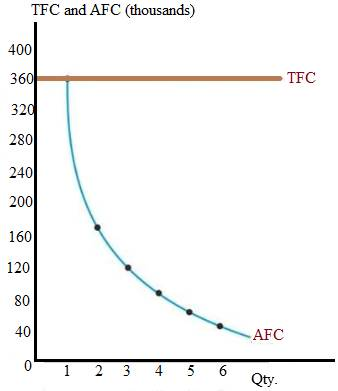# A firm’s total fixed cost is $360,000. Construct a table of its total and average fixed costs for output levels varying from zero to six units. Draw the corresponding TFC and AFC curves.BuyFind ### Microeconomics: Principles & Policy 14th Edition William J. Baumol + 2 others Publisher: Cengage Learning ISBN: 9781337794992BuyFind ### Microeconomics: Principles & Policy 14th Edition William J. Baumol + 2 others Publisher: Cengage Learning ISBN: 9781337794992 #### Solutions Chapter 7, Problem 1TY Textbook Problem ## A firm’s total fixed cost is$360,000. Construct a table of its total and average fixed costs for output levels varying from zero to six units. Draw the corresponding TFC and AFC curves.

Expert Solution
To determine

To find: The table showing TFC and AFC and the curve of these costs.

### Explanation of Solution

Below is the complete table. Here, the average fixed cost is calculated by dividing the total fixed cost by outputs.

AFC=TFCQ

 Output Total Fixed cost (fig. in thousands) AFC (fig. in thousands) 0 360 0 1 360 360 2 360 180 3 360 120 4 360 90 5 360 72 6 360 60

Below is the curve that shows the total fixed cost curve and the average fixed cost curve. Here, the horizontal axis shows the output. While the vertical axis shows the price or costs.### Want to see more full solutions like this?

Subscribe now to access step-by-step solutions to millions of textbook problems written by subject matter experts!

### Want to see more full solutions like this?

Subscribe now to access step-by-step solutions to millions of textbook problems written by subject matter experts!

## Chapter 7 Solutions

Microeconomics: Principles & Policy